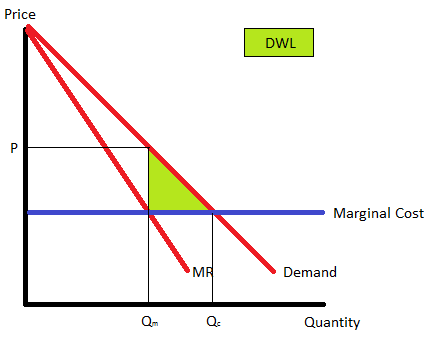BackAP 各学科「速成」笔记导览

# Unit 1: Basic Economic Concept

## Opportunity Cost

• $$\text{Opportunity Cost} = \frac{\text{forgone}}{\text{gain}}$$

• Possibility Production Frontier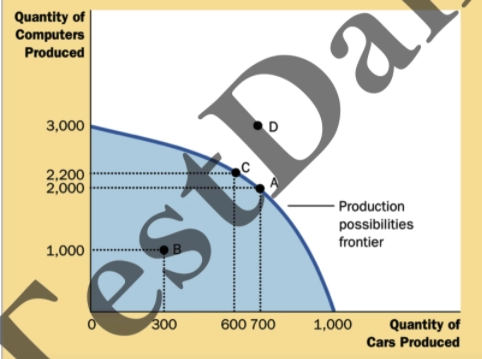### Shift of OC

• Point Shift
• Inside the Frontier
• Input resources do not be allocated efficiently
• Unemployment
• On the Frontier
• Resources have been allocated efficiently
• Outside the Frontier
• We can comsume by importing goods
• Impossible
• Curve shift
• X Tech increase → X Border shift outward
• No effect
• Demand of product
• Price of product
• Other factor that don’t do with allocating efficiency

### Shape of OC

• Bowed outward / convex

• Increasing OC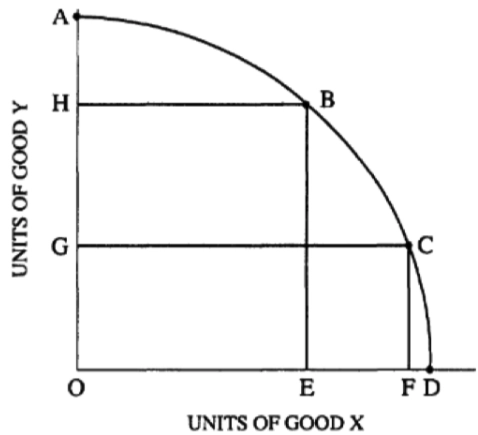• Bowed in / concave

• Decreasing OC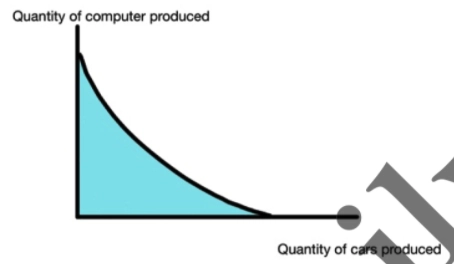• Straight Line

• Constant OC
• Resources are completely adaptable to alternative uses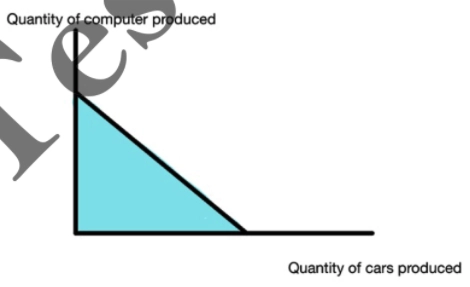• AP Classroom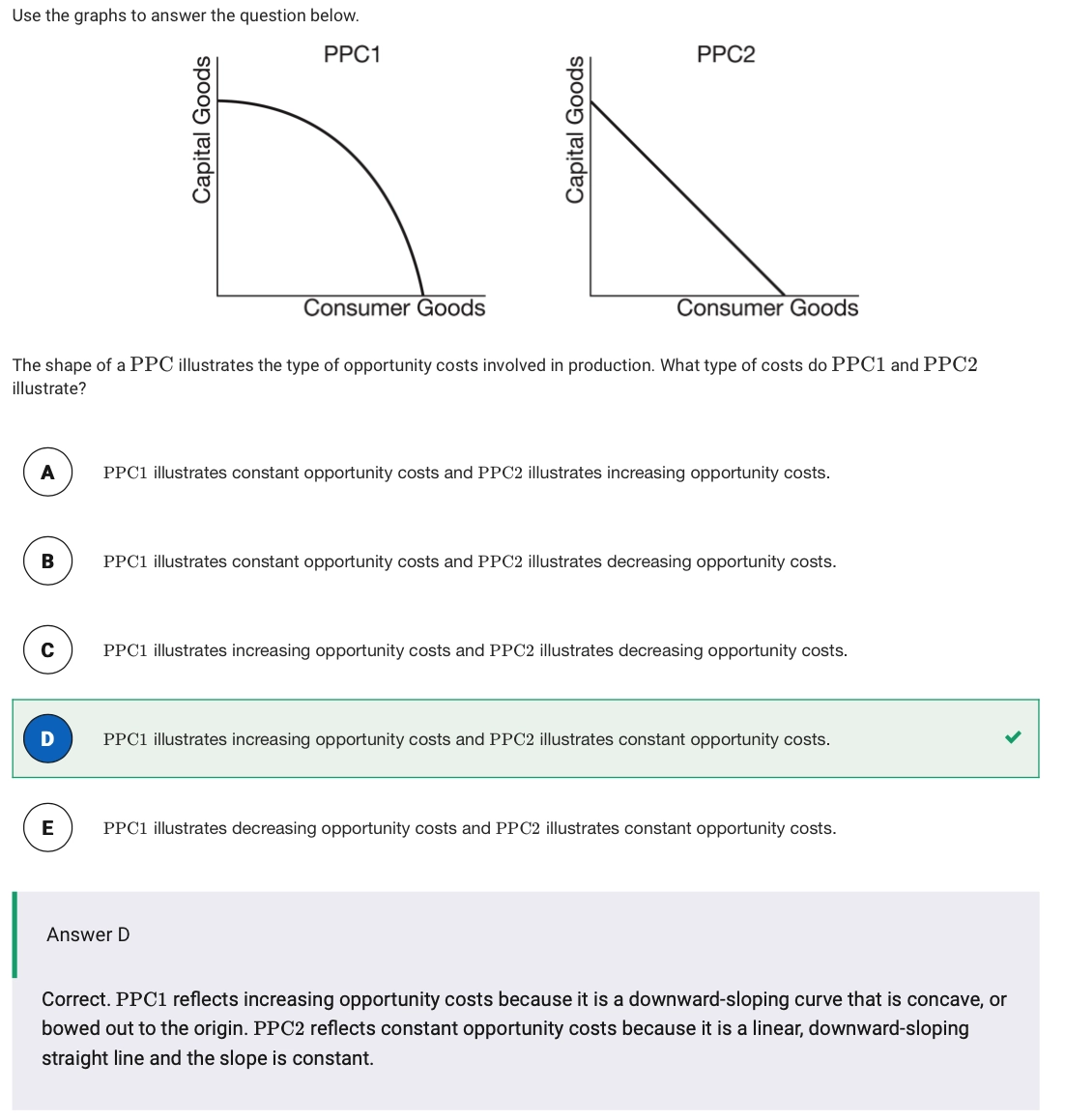• Someone using same input could absolutely gain greater amount of some product than others.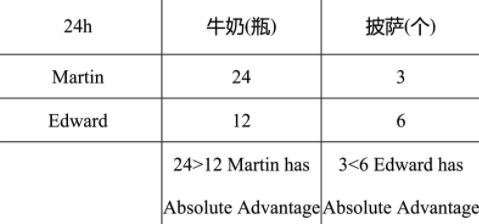• Someone using same input could relatively / comparatively have lower opportunity cost than others.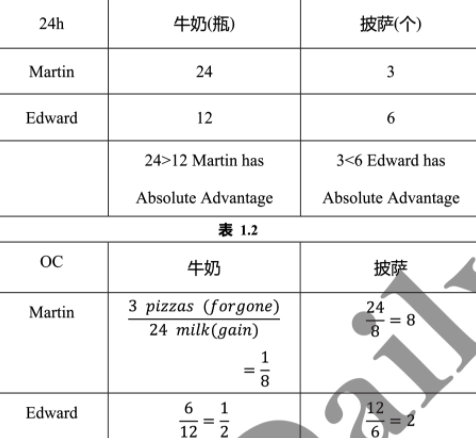• Milk: Martin
• Pizza: Edward
• Input question: $$N$$ hours → produce $$1$$ unit
• Output question: $$24\text{(or arbitrary fixed hours)}$$ -> $$N$$ unit
• Generally, we transform the input question to output question through a fixed input hours. (e.g $$24$$ hours)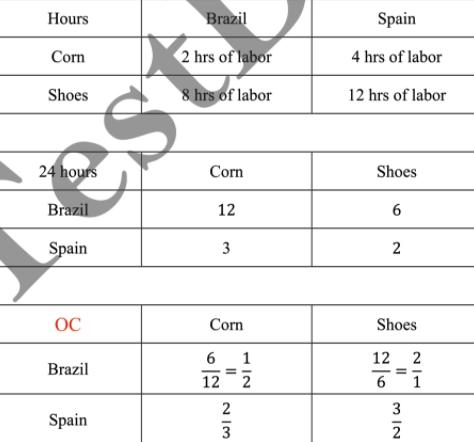• If countries trade based on the opportunity cost, country A would uses one unit product A to exchange a range amount of product B between country A OC of product A to country B OC of product A.

Untitled

• Price of product A will be a range between $$2 \sim 3$$

## Utility

• Maximizing Utility Formula

$\frac{\text{MU}_x}{\text{P}_x} = \frac{\text{MU}_y}{\text{P}_y}$

• Double income, price
• No effect

# Unit 2: Supply and Demand

## Elasticity

### General Formula

$\text{Elasticity} = \frac{\Delta\text{Quantity}\%}{\Delta\text{Price}\%}$

### Change rate (Take $$\Delta Q$$ as an example)

• Divide original amount of quantity

• A to B

$\Delta \text{Quantity}\% = \frac{Q_B - Q_A}{Q_A}$

• B to A

$\Delta \text{Quantity}\% = \frac{Q_A - Q_B}{Q_B}$

• Mid-point

$\Delta \text{Quantity}\% = \frac{Q_A - Q_B}{\frac{Q_A + Q_B}{2}}$

### Elasticity of Curve

• tend to be even / horizontal curve
• more elasticity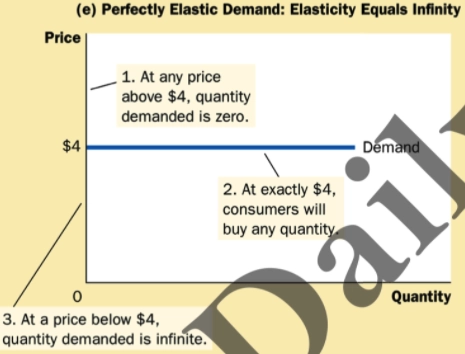• tend to be steep / vertical curve
• more inelasticity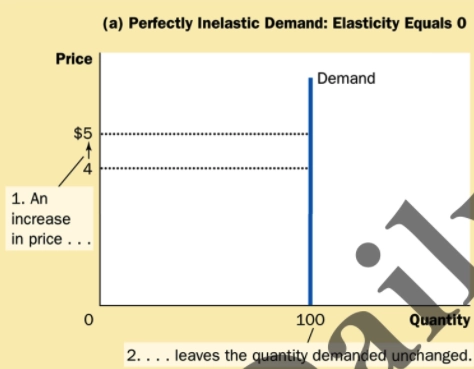### TR & Elasticity

• As shown in the following figure: Firms usually operate in $$\text{Elasticity} > 0$$ since $$MR > 0$$, $$TR$$ is continuously increasing.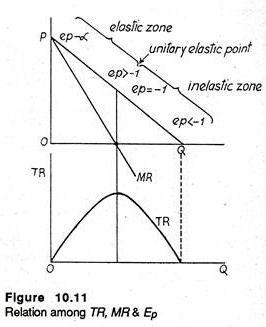### Income Elasticity of Demand (IED)

$\text{IED} = \frac{\Delta\text{Quantity}\%}{\Delta \text{Income}\%}$

• $$\text{IED} > 0$$, which means $$\Delta Q,\;\Delta I$$ changing toward same direction. Normal Good (+)
• $$\text{IED} < 0$$, which means $$\Delta Q,\;\Delta I$$ changing toward opposite direction. Inferior Good (-

### Cross-price Elasticity of Demand (XED)

$\text{XED} = \frac{\Delta\text{Quantity}_X\%}{\Delta \text{Price}_Y\%}$

• $$\text{XED} > 0$$, which means $$\Delta Q_X,\;\Delta P_Y$$ changing toward same direction. Substitutes (+)
• $$\text{XED} < 0$$, which means $$\Delta Q_X.\;\Delta P_Y$$ changing toward opposite direction. Complement (-)

## Efficiency

### Allocated Efficiency

• Maximize the Total Surplus, $$\text{S = D}$$
• Example
• Market equilibrium
• Supply = Demand
• Perfectly Competitive Market MC (Supply) = MR (Demand)

### Production Efficiency

• Production Efficiency is Minimize the Production Cost
• Example:
• ATC intersects MC

## Tax

### Per-unit Tax

• Per-unit Tax: Size of Tax
• For Example:
• Per-unit Tax is $$\3.30 - \2.80 = \0.5$$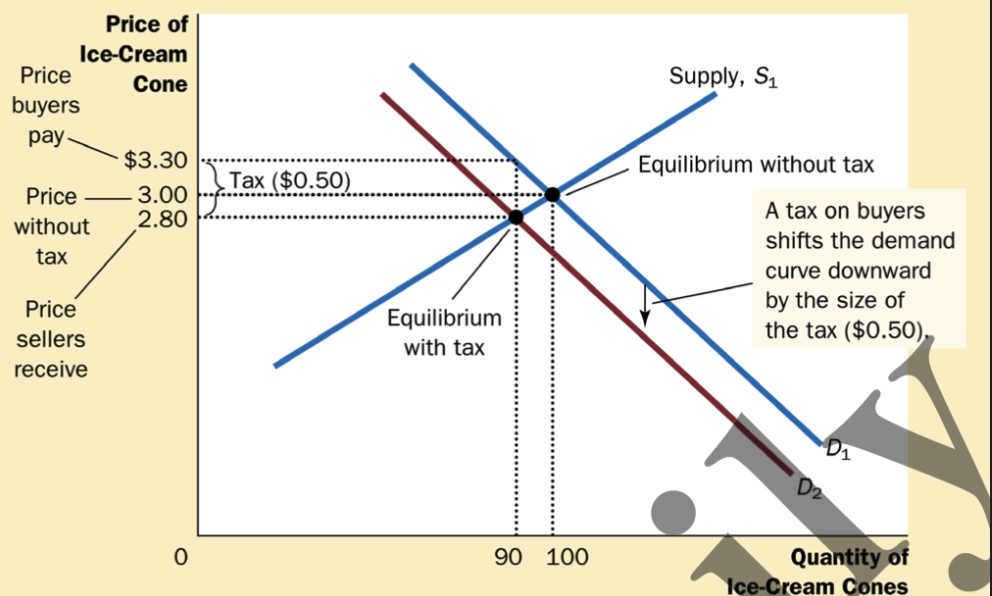### Tax and Elasticity

• Which curve is more elastic, the less tax they need to pay.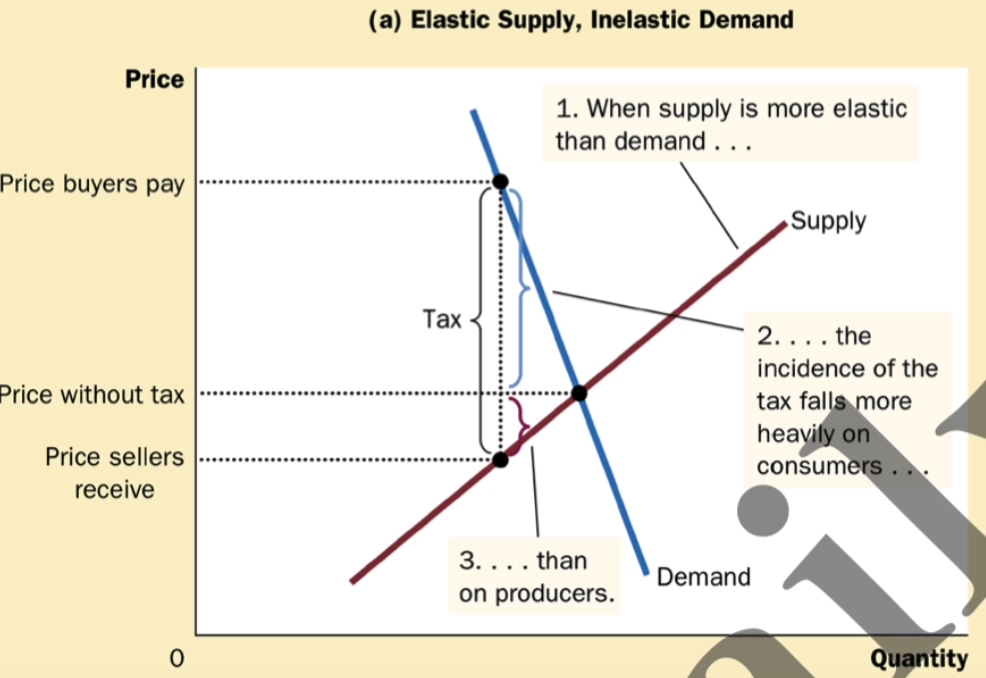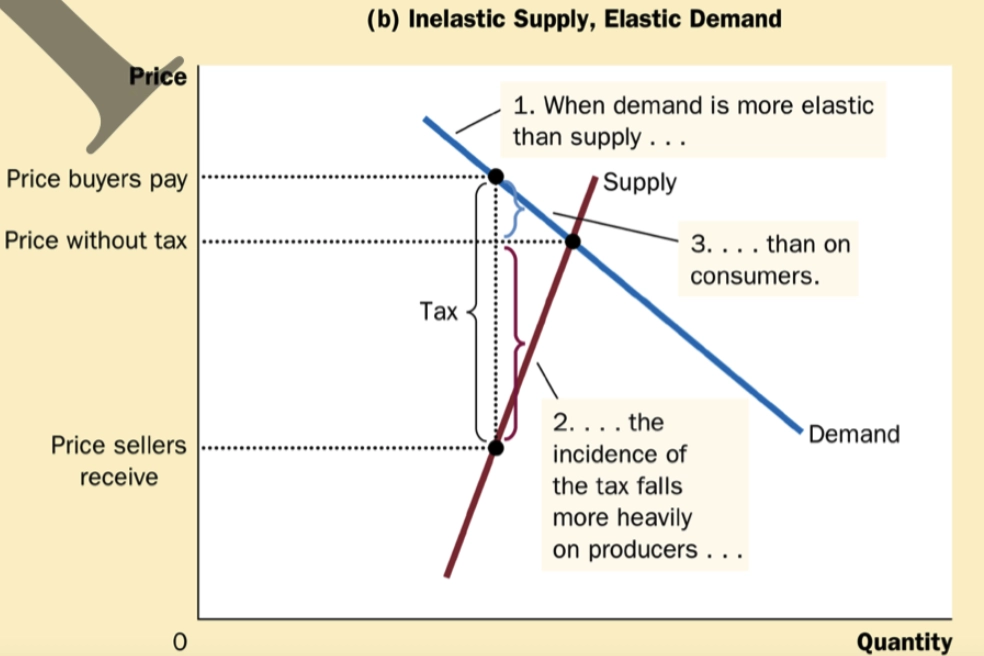• The surplus that consumer surplus and producer surplus could not reach.

$\text{Deadweight Loss} = \text{Total Surplus} - (\text{Comsumer Surplus} + \text{Producer Surplus})$

• Government Action

• Price Ceiling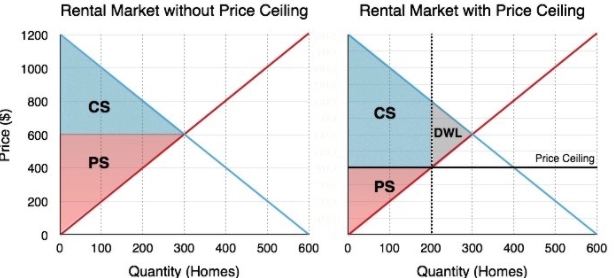• Price Floor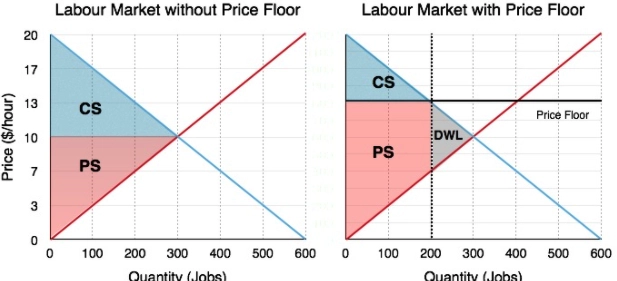• Tax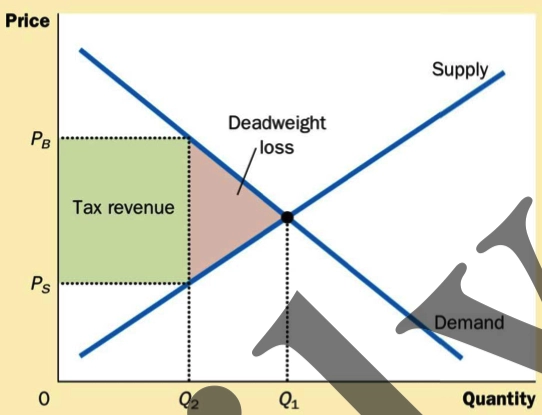• Tariff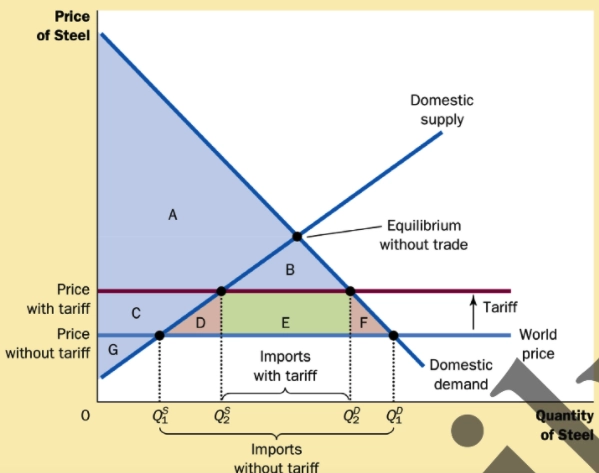• Subsidy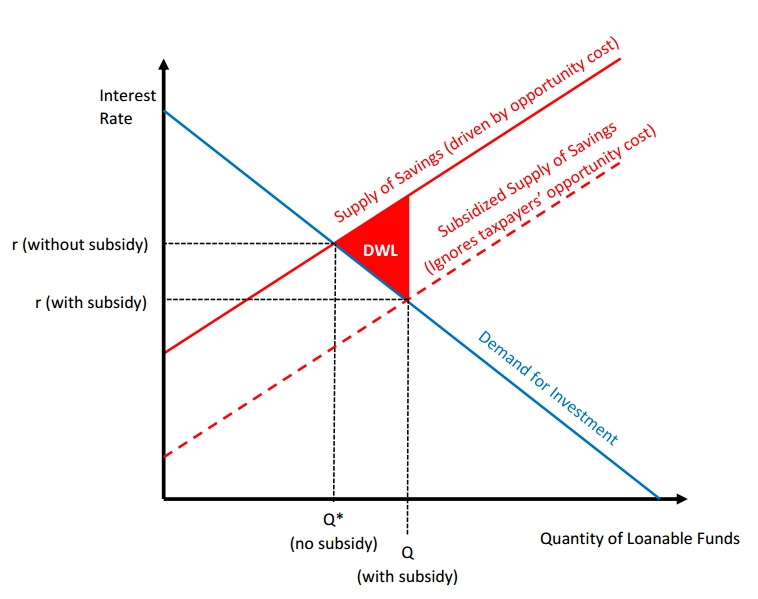• Monopoly